Christoffel symbols, Levi-Civita connection

The Levi-Civita connection involves the Christoffel (1,2)-tensor field, which is implemented by the command ΜΓ. Assume our metric is that of the geometrical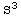, parametrized in spherical coordinates asThen, the Christoffel (1,2)-tensor field is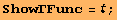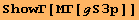Choosing slightly different coordinates results in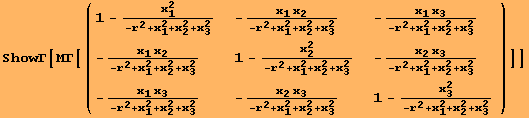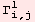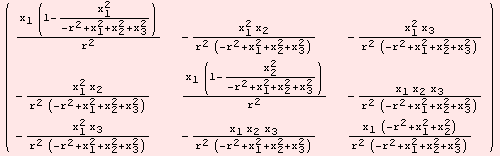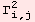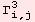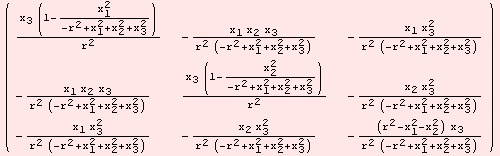The Levi-Civita connection is equivalent to a special covariant derivative, which we implement by Μ▽ in a brief way.Global`Μ▽

 Μ▽[g_,X_List,r_Integer:1]:=Μd[X,r]+Τdα[X,r,ΜΓ[g]]

The command Μ▽ determines the covariant derivative of tensor fields of arbitrary rank. Below, we compute the covariant derivative of the vector field {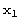,,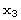}. The result is a (1,1)-tensor field.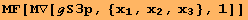Created by Mathematica  (December 22, 2006)